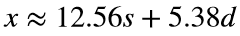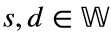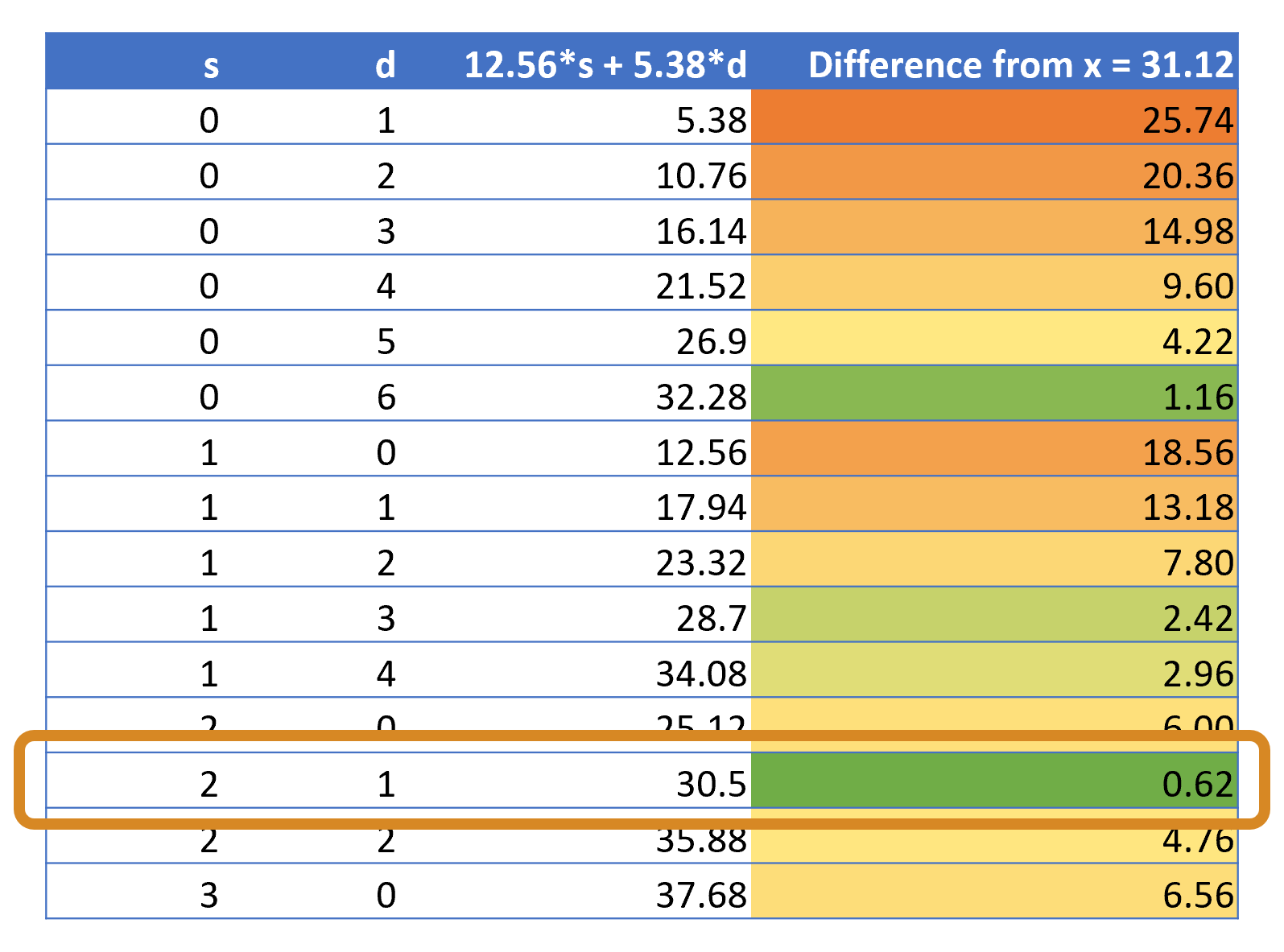# Problem 56508. Cricket - Represent Average in "Dulkars" and "Ses"

Sachin Tendulkar's Test average was 53.78. So if Tendulkar = 53.78, one dulkar = 5.378. Similarly, Roger Twose's average was 25.12. So if Twose = 25.12, one se = 12.56.
Given a batter's average x, return a 2-element vector v = [s d] that represents the number of whole (integer) units of Ses (12.56) and Dulkars (5.38) that most closely represents x. That is,, where.
For example, if x = 31.12, v = [2 1]:### Solution Stats

45.76% Correct | 54.24% Incorrect
Last Solution submitted on Nov 02, 2023